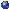Symmetric tensors are multi-dimensional arrays invariant to permutation of indices. Symmetric tensors arise in data science when applying the method of moments, as higher-dimensional analogs of the sample covariance matrix. In tasks from demixing Gaussian mixture models to blind source separation, it is informative to decompose a symmetric tensor as a sum of symmetric outer products of vectors. This talk presents a novel algorithm for computing low-rank symmetric tensor decompositions, based on a modified tensor power method. Numerical experiments demonstrate that our algorithm significantly outperforms state-of-the-art methods, per standard performance metrics. We provide supporting theoretical guarantees, through connections to optimization theory, algebraic geometry and dynamical systems. We also extend the algorithm to compute a certain generalization of symmetric tensor decompositions. By applying the method of moments, this enables estimation of a union of linear subspaces from noisy point samples, i.e., robust subspace clustering. Applications to motion segmentation and image segmentation are discussed. Finally, parallels to numerical linear algebra motivate a plethora of interesting open problems.